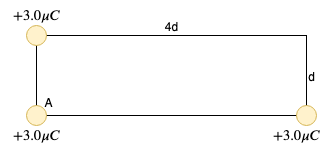# In the rectangle in the drawing, a charge is to be placed at the empty corner to make the net...

## Question:

In the rectangle in the drawing, a charge is to be placed at the empty corner to make the net force on the charge at corner A point along the vertical direction. What change (magnitude and algebraic sign) must be placed at the empty corner?Number _____ units _____

the tolerance is +/- 2%

## Electric Force:

Coulomb's law states that the magnitude of the force between two point charges is directly proportional to the product of the magnitude of the two charges and inversely proportional to the square of their separation.

Become a Study.com member to unlock this answer! Create your account

The magnitude of the electric force between two point charges Q and q separated by a distance r is given by the expression

F \ = \ k \ \frac{|Q| \...Electric Force: Definition & Equation

from

Chapter 14 / Lesson 12
172K

The electric force is one of the most powerful, fundamental forces in the universe. In this lesson, we will learn how charged objects exert electric forces on each other and how to calculate the strength of that force.